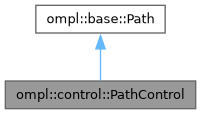ompl::control::PathControl Class Reference

Definition of a control path. More...

#include <ompl/control/PathControl.h>

Inheritance diagram for ompl::control::PathControl:[legend]

## Public Member Functions

PathControl (const base::SpaceInformationPtr &si)
Constructor.

PathControl (const PathControl &path)
Copy constructor.

PathControloperator= (const PathControl &other)
Assignment operator.

base::Cost cost (const base::OptimizationObjectivePtr &opt) const override
Not yet implemented.

double length () const override
The path length (sum of control durations)

bool check () const override
Check if the path is valid.

void print (std::ostream &out) const override
Print the path to a stream.

virtual void printAsMatrix (std::ostream &out) const
Print the path as a real-valued matrix where the i-th row represents the i-th state along the path, followed by the control and duration needed to reach this state. For the first state the control and duration are zeroes. The state components printed are those returned by ompl::base::StateSpace::copyToReals, while the control components printed are the discrete components (if any) followed by the real-valued ones as returned by ompl::control::ControlSpace::getValueAddressAtIndex.

geometric::PathGeometric asGeometric () const
Convert this path into a geometric path (interpolation is performed and then states are copied)

Path operations
void append (const base::State *state)
Append state to the end of the path; it is assumed state is the first state, so no control is applied. The memory for state is copied. There are no checks to make sure the number of controls and states make sense.

void append (const base::State *state, const Control *control, double duration)
Append state to the end of the path and assume control is applied for the duration duration. The memory for state and for control is copied. There are no checks to make sure the number of controls and states make sense.

void interpolate ()
Make the path such that all controls are applied for a single time step (computes intermediate states)

void random ()
Set this path to a random segment.

bool randomValid (unsigned int attempts)
Set this path to a random valid segment. Sample attempts times for valid segments. Returns true on success.

Functionality for accessing states and controls
std::vector< base::State * > & getStates ()
Get the states that make up the path (as a reference, so it can be modified, hence the function is not const)

std::vector< Control * > & getControls ()
Get the controls that make up the path (as a reference, so it can be modified, hence the function is not const)

std::vector< double > & getControlDurations ()
Get the control durations used along the path (as a reference, so it can be modified, hence the function is not const)

base::StategetState (unsigned int index)
Get the state located at index along the path.

const base::StategetState (unsigned int index) const
Get the state located at index along the path.

ControlgetControl (unsigned int index)
Get the control located at index along the path. This is the control that gets applied to the state located at index.

const ControlgetControl (unsigned int index) const
Get the control located at index along the path. This is the control that gets applied to the state located at index.

double getControlDuration (unsigned int index) const
Get the duration of the control at index, which gets applied to the state at index.

std::size_t getStateCount () const
Get the number of states (way-points) that make up this path.

std::size_t getControlCount () const
Get the number of controls applied along this path. This should be equal to getStateCount() - 1 unless there are 0 states, in which case the number of controls will also be 0.Public Member Functions inherited from ompl::base::Path
Path (const Path &)=delete

Pathoperator= (const Path &)=delete

Path (SpaceInformationPtr si)
Constructor. A path must always know the space information it is part of.

virtual ~Path ()=default
Destructor.

const SpaceInformationPtrgetSpaceInformation () const
Get the space information associated to this class.

template<class T >
const T * as () const
Cast this instance to a desired type. More...

template<class T >
T * as ()
Cast this instance to a desired type. More...

virtual Cost cost (const OptimizationObjectivePtr &obj) const =0
Return the cost of the path with respect to a specified optimization objective.

## Protected Member Functions

void freeMemory ()
Free the memory allocated by the path.

void copyFrom (const PathControl &other)
Copy the content of a path to this one.

## Protected Attributes

std::vector< base::State * > states_
The list of states that make up the path.

std::vector< Control * > controls_
The control applied at each state. This array contains one element less than the list of states.

std::vector< double > controlDurations_
The duration of the control applied at each state. This array contains one element less than the list of states.Protected Attributes inherited from ompl::base::Path
SpaceInformationPtr si_
The space information this path is part of.

## Detailed Description

Definition of a control path.

This is the type of path produced when planning with differential constraints.

Definition at line 92 of file PathControl.h.

The documentation for this class was generated from the following files: